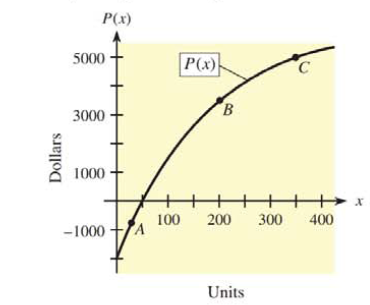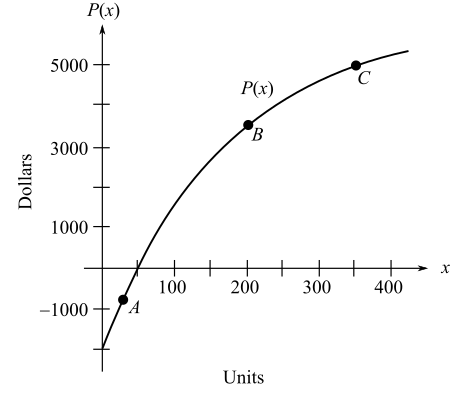Chapter 9.9, Problem 31E### Mathematical Applications for the ...

11th Edition
Ronald J. Harshbarger + 1 other
ISBN: 9781305108042

#### Solutions

Chapter
Section### Mathematical Applications for the ...

11th Edition
Ronald J. Harshbarger + 1 other
ISBN: 9781305108042
Textbook Problem

# In each of Problems 31 and 32, the graph of a company’s profit function is shown. For each problem, use the graph to answer the following questions about points A, B, and C.(a) Rank from smallest to largest the amounts of profit received at these points. Explain your choices, and note whether any point results in a loss.(b) Rank from smallest to largest the marginal profit at these points. Explain your choices, and note whether any marginal is negative and what this means.(a)

To determine

The profit in smallest to largest order at these points A,B,C from the provided graph and find out any point of loss.Explanation

Given information:

The provided graph is:

Explanation:

The provided graph is between profit and unit.

The graph is increasing order from A to C

The profit is increased as number of units increased.

Therefore, from the graph the lowest profit is at A and it is a loss point because the profit is negative at this point

(b)

To determine

The marginal profit in smallest to largest order at these points A,B,C from the provided graph and find out the point of negative marginal.### Still sussing out bartleby?

Check out a sample textbook solution.

See a sample solution

#### The Solution to Your Study Problems

Bartleby provides explanations to thousands of textbook problems written by our experts, many with advanced degrees!

Get Started

#### Find more solutions based on key concepts